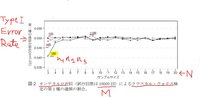# Monte Carlo Convergence

#### fahdguthmy

##### New member
I was able to perform a monte Carlo simulation to estimate the p-value of a krusal-wallis test using emissions data of 500 motor vehicles.
Now my professor wants me to show how the p-value converges as N increases from a small number to a larger number. He asked me to start with a sample size (N) of 3 and increase to 20 and plot N on the x-axis and type 1 error on the y-axis like this:N=sample size
M= number of trials
lines represent the treatments/groups (i.e. 3, 4, 5 and 10 groups).

For N=3...20, I picked N random vehicle emissions from the population of 500 cars and performed each of the 18 simulations. However, there is absolutely no convergence when it comes to the p-values... I don't see how there would even be a convergence to the population p-value using such small sample sizes even if you use 100,000 trials!
Where could I be messing up?